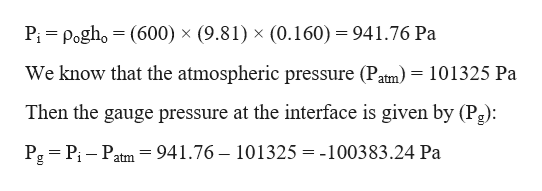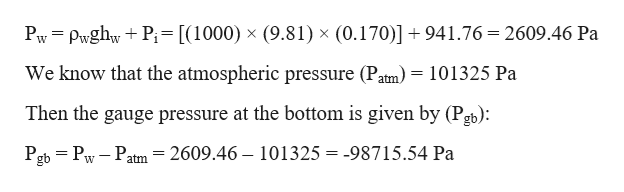# A barrel contains a 0.160 m layer of oil floating on water that is 0.170 m deep. The density of the oil is 600 kg/m3.a. What is the gauge pressure at the oil-water interface?b. What is the gauge pressure at the bottom of the barrel?

Question
125 views

A barrel contains a 0.160 m layer of oil floating on water that is 0.170 m deep. The density of the oil is 600 kg/m3.

a. What is the gauge pressure at the oil-water interface?

b. What is the gauge pressure at the bottom of the barrel?

check_circle

Step 1

Given information:

Height of water level in the barrel (hw) = 0.170 m

Height of oil level above the water-oil interface (ho) = 0.160 m

Density of the oil (ρo) = 600 kg/m3

Density of water (ρw) = 1000 kg/m3

Step 2

Pressure at the water-oil interface (Pi):help_outlineImage TranscriptionclosePi Pogho (600) x (9.81) x (0.160) = 941.76 Pa We know that the atmospheric pressure (Patm) =101325 Pa Then the gauge pressure at the interface is given by (Pg): Pg Pi Patm= 941.76 - 101325 = -100383.24 Pa fullscreen
Step 3

Pressure at the bottom of the w...help_outlineImage TranscriptionclosePw PwghwP= [(1000) x (9.81) x (0.170)] + 941.76 = 2609.46 Pa We know that the atmospheric pressure (Patm) = 101325 Pa Then the gauge pressure at the bottom is given by (Pgb): 2609.46 Pgb Pw Patm 101325 = -98715.54 Pa fullscreen

### Want to see the full answer?

See Solution

#### Want to see this answer and more?

Solutions are written by subject experts who are available 24/7. Questions are typically answered within 1 hour.*

See Solution
*Response times may vary by subject and question.
Tagged in

### Fluid Mechanics